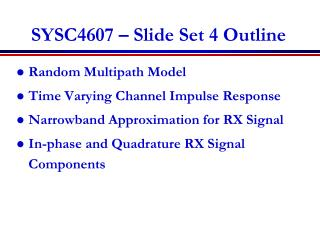DownloadDownload PresentationSYSC4607 – Slide Set 4 Outline

SYSC4607 – Slide Set 4 Outline

Télécharger la présentationSYSC4607 – Slide Set 4 Outline

- - - - - - - - - - - - - - - - - - - - - - - - - - - E N D - - - - - - - - - - - - - - - - - - - - - - - - - - -
Presentation Transcript

1. SYSC4607 – Slide Set 4 Outline • Random Multipath Model • Time Varying Channel Impulse Response • Narrowband Approximation for RX Signal • In-phase and Quadrature RX Signal Components

2. Statistical Multipath Model • Random # of multipath components, each with • Random amplitude • Random phase • Random Doppler shift • Random delay • Random components change with time • Leads to time-varying channel impulse response

3. Time Varying Impulse Response • Response of channel at t to impulse at t-t: • t is time when impulse response is observed • t-t is time when impulse put into the channel • t is how long ago impulse was put into the channel for the current observation

4. Received Signal Characteristics • Received signal consists of many multipath components • Amplitudes change slowly • Phases change rapidly • Constructive and destructive addition of signal components • Amplitude fading of received signal (both wideband and narrowband signals)

5. Narrowband Model • Assume delay spread maxm,n|tn(t)-tm(t)|<<1/B (nonresolvable multipath components) • Then u(t)u(t-t). • Received signal given by • No signal distortion (spreading in time) • Multipath affects complex scale factor in brackets. • Characterize scale factor by setting u(t)=exp(jφ0), whereφ0is a fixed random phase, i.e., s(t)=cos(2πfct+φ0)

6. In-Phase and Quadratureunder CLT Approximation • In phase and quadrature signal components: • For N(t) large, rI(t) and rQ(t) jointly Gaussian (sum of large # of independent random variables). • Received signal is also Gaussian characterized by its mean, and autocorrelation function. • If jn(t) uniform, the in-phase/quad components are zero-mean and jointly WSS with the same autocorrelation function. • Received signal is also WSS.

7. Main Points • Statistical multipath model leads to a time-varying channel impulse response • Received signal has random amplitude fluctuations • Narrowband model and CLT lead to in-phase/quad components that are stationary Gaussian processes • Processes completely characterized by their mean, autocorrelation, and cross correlation. • Assuming uniform phase offsets, the received process is a zero mean Gaussian process.